#

# 1.介绍

• 1）低延迟、高吞吐，共享主要内存：这样的系统中一个处理器可以以超过12GB/s的速率读写共享物理内存，延迟只有数10ns
• 2）跨多磁盘高带宽（high bandwidth off multiple disks）：一个上千美金的RAID可以以超过1GB/s的速率将数据导入到主存中。而作为对比，由于存在容错机制上频繁的checkpoint，一个典型的MapReduce setup会以低于数十MB/s的速率读取数据。多核系统可以达到高速率，瓶颈在处理器间的同步（或locking）上。因而，为了能在多核机器上允许可扩展的数据分析，一些好的解决方案必须能最小化加锁开销。

# 2.稀疏可分的cost functions

…(1)

(2.1)的cost function引入了一个hypergraph：$G=(V,E)$，它的节点是x的单个元素。每个子向量$x_e$相当于在图$e \in E$中引入一条边，它包含了节点的一些子集。以下的示例展示了该概念。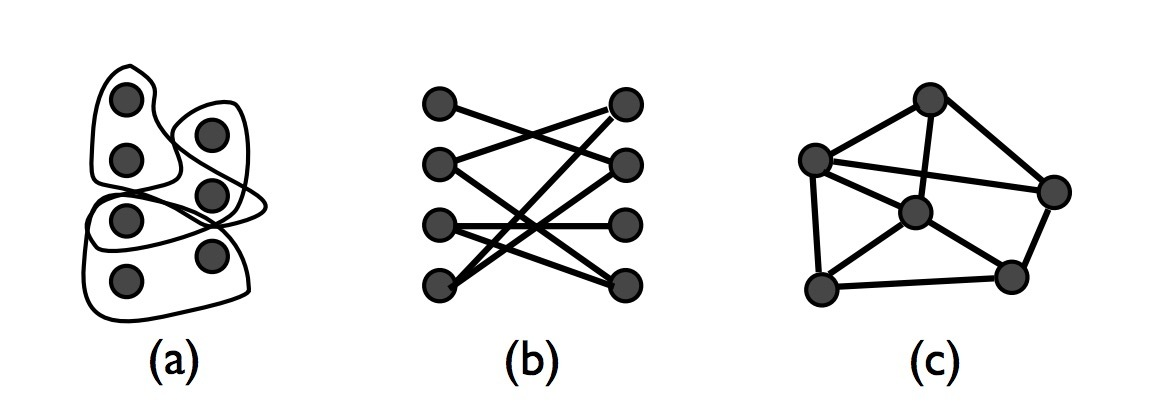…(2.2)

…(2.3)

…(2.4)

## 2.4 结论

…(2.6)

$\Omega$可以简单量化hyper edges的size。$\rho$决定了与任意给定edge交叉的edges最大比例。$\Delta$决定了与任何变量交叉的edges的最大比例。$\rho$是一个关于hypergraph稀疏度的衡量，其中$\Delta$决定着节点的正则化（node-regularity）。对于我们的例子，我们可以做出以下关于$\rho$$\Delta$的观察：

• 1.Sparse SVM。$\Delta$是在一个样本中出现的任何特征的最大频率，$\rho$决定着hypergraph是如何聚类的。如果一些特征在整个数据集上很常见，那么$\rho$会与某一个很接近。
• 矩阵补全（Matrix Completion）：如果我们假设，提供的样本是随机均匀抽样的，我们可以看到超过$n_c log(n_c)$个样本，接着$\Delta \approx \frac{log(n_r)}{n_r}$$\rho \approx \frac{2log(n_r)}{n_r}$ 。它会遵从一个彩票收集问题（coupon collector argument）。
• 3.Graph Cuts。 $\Delta$是由$\mid E \mid$划分的最大度(degree)，$\rho$至少为$2\Delta$

# 3.Hogwild!算法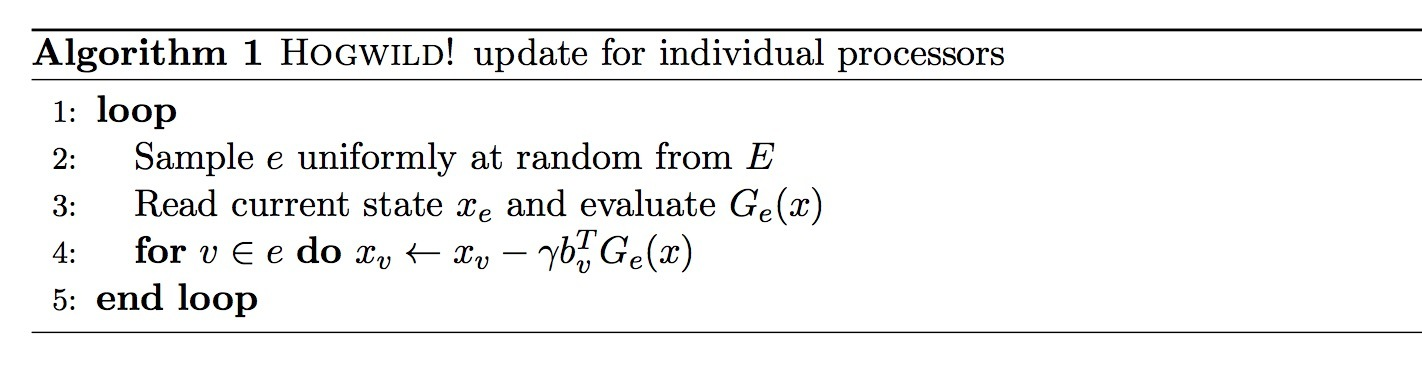• $b_v$表示在$R^n$中的标准基元素（standard basis elements）之一，其中v的范围为1, …, n。也就是说，$b_v$在第v个component上等于1, 否则为0. 假设$P_v$表示在坐标v上的欧几里德投影矩阵，例如：$P_v = b_v b_v^T$。其中$P_v$是一个对角矩阵，它在第v个对角上为1,否则为0。
• $G_e(x) \in R^n$表示函数$f_e$$\mid E \mid$相乘的一个梯度或者子梯度。也就是说，我们将$f_e$，从坐标e上的一个函数扩展为$R^n$上所有坐标上，通过忽略下标e来得到。那么：

(3.1)

• $\gamma$: 我们假设，stepsize $\gamma$是一个固定的常数。
• $x_j$: 尽管这些处理器并不知道任何其它处理器是否已经修改了x，我们定义$x_j$是决策变量x在j执行更新后的状态(state)。由于两个处理器可能会在同时写入x，我们需要注意该定义，但我们会通过随机方式来简单断绝关系。注意，$x_j$通常使用一个旧的梯度进行更新，它基于x的一个在多个时钟周期前读取的值。我们使用$x_{k(j)}$来表示用于产生状态$x_j$的用于计算梯度或次梯度的决策变量值。

…(4.1)

…(4.2)

…(4.3)

…(4.4)

…(4.5)

…(4.6)

# 5.Robust 1/k rates

…(5.1)

$\gamma$上的依赖，对于下面的情况很有用。将(5.1)化解开：

…(5.2)

…(5.3)

(5.4)

k轮迭代后足够收敛到该球体。注意，这是一个线性rate的收敛率。

## 5.1 顺序型（serial）SGD的结论

的作者演示了一个大的step size: $\gamma_k = \frac{\theta}{2ck}, \theta > 1$ 可以产生一个边界：

$\beta \approx 0.37$是最小的，等于1.34. 表达式：

$\theta=2$时是最小的，等于1. 因此，产生的常数在constant stepsize protocol中当所有其它参数设置为最优时，会稍微变差些。然而，如果$D_0 \gt M^2/c^2$，那么1/k protocol的error会与$D_0$成比例，但我们的constant stepsize protocol仍在初始距离上只有一个log依赖。再者，constant stepsize scheme在对曲率参数c高估上会更健壮些（robust）。而对于1/k protocol，如果你高估了曲率（对应于$\theta$的一个小值），你会获得较慢的收敛率。简单的，在中的一维样本展示了，$\theta=0.2$可以生成一个$k^{-1/5}$的收敛率。在我们的scheme中，$\vartheta=0.2$会通过一个因子5来简单增加迭代数目。

# 7.实验

Sparse SVM。我们在Reuters RCV1数据集上测试了我们的sparse SVM实现，使用二分类任务CCAT。它具有804414条样本，划分为：23149训练样本，781265测试样本，存在47236个特征。我们在我们的实验中交换了训练集和测试集，以演示并行多核算法的可扩展性。在本例上，$\rho = 0.44, \Delta = 1.0$，Hogwild中大值通常会产生一个badcase。在图3a中，我们看到，Hogwild可以达到3倍加速，而RR会随着线程的增加而变差。确实，对于快速梯度，RR要比顺序实现（serial implement）还差。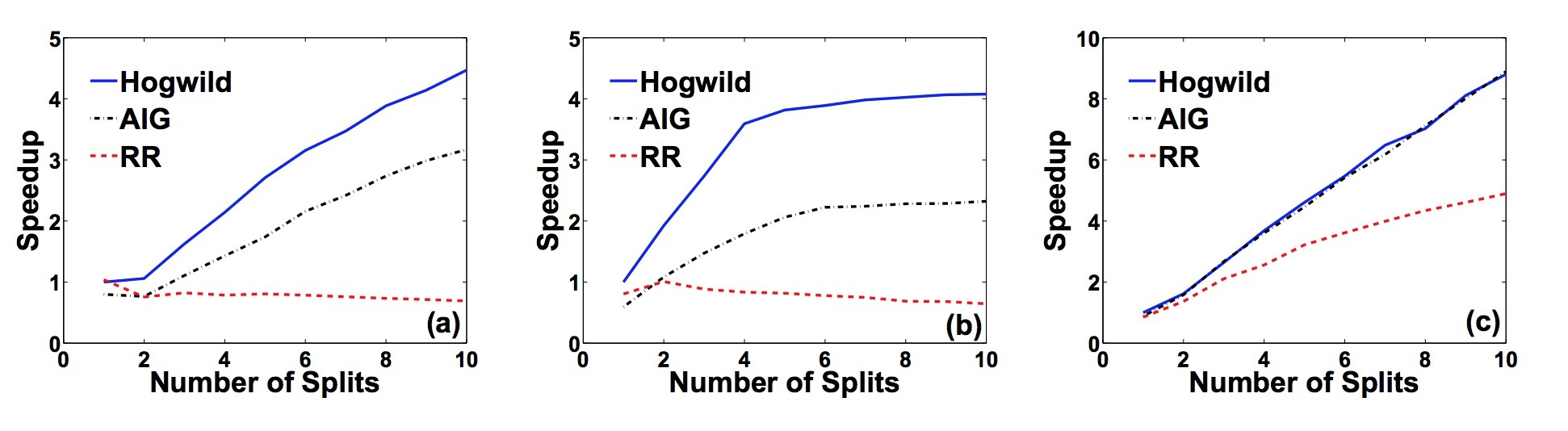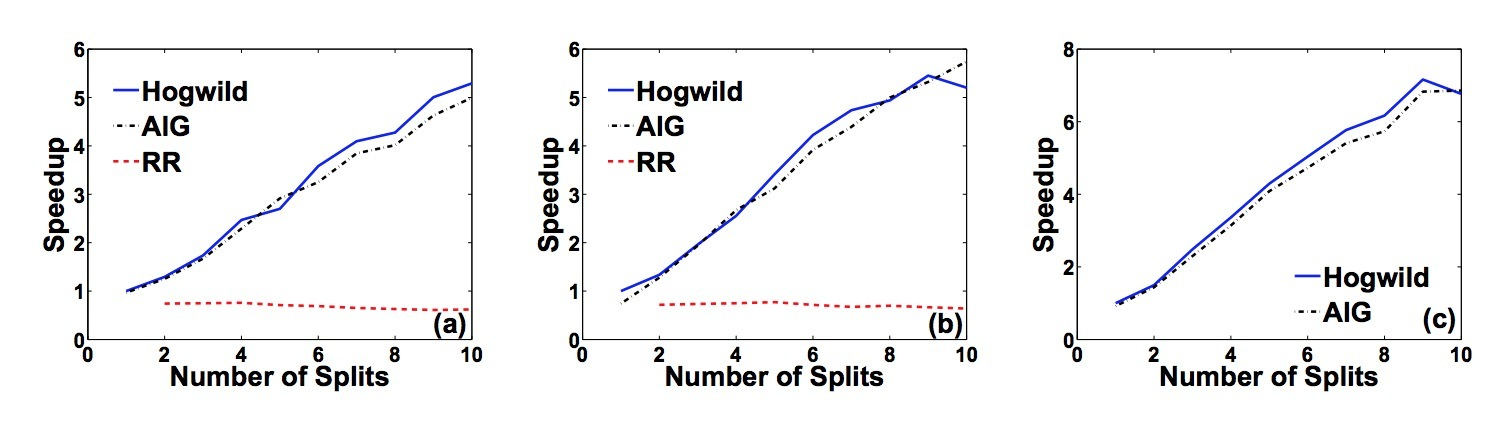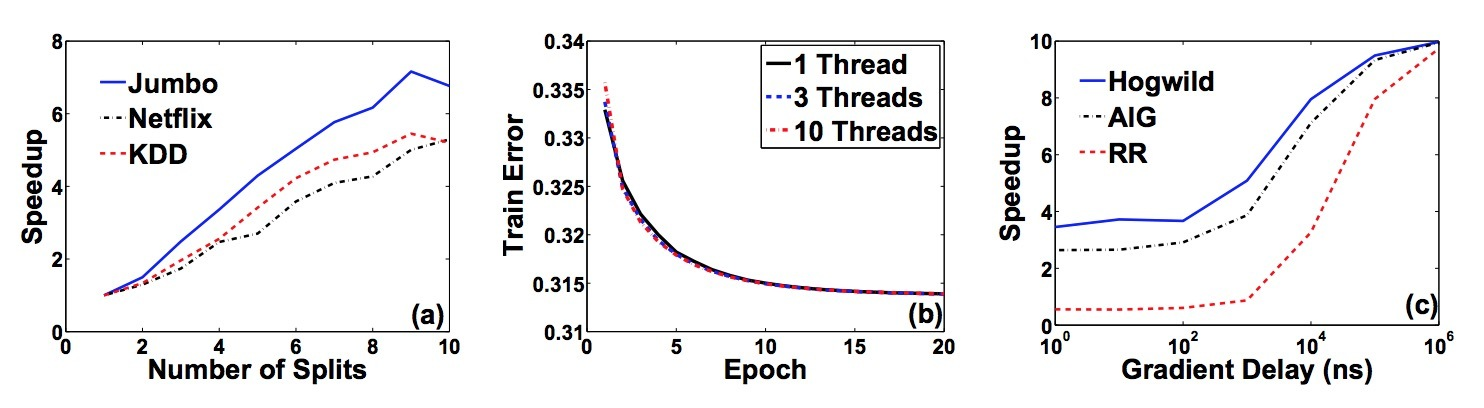Graph Cuts。 我们的第一个cut问题是一个在计算机视觉中很流行的标准图片切分。xxx（略）

Updated on